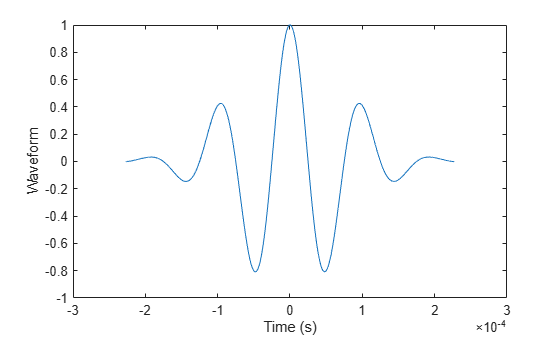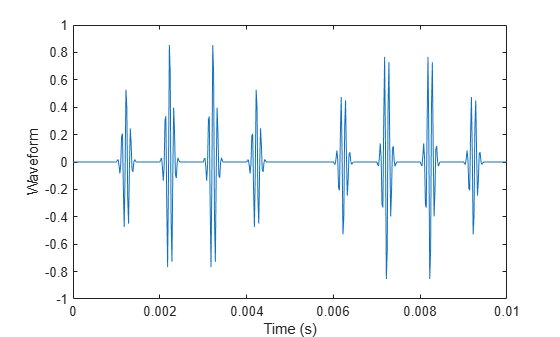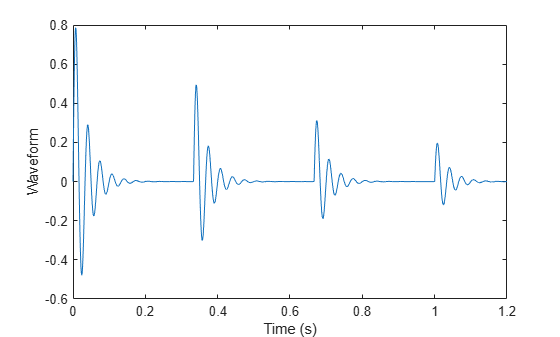# pulstran

## Syntax

``y = pulstran(t,d,func)``
``y = pulstran(t,d,func,fs)``
``y = pulstran(t,d,p)``
``y = pulstran(___,intfunc)``

## Description

example

````y = pulstran(t,d,func)` generates a pulse train based on samples of a continuous function, `func`.```

example

````y = pulstran(t,d,func,fs)` uses a sample rate of `fs`.```

example

````y = pulstran(t,d,p)` generates a pulse train that is the sum of multiple delayed interpolations of the prototype pulse in vector `p`.```

example

````y = pulstran(___,intfunc)` specifies alternative interpolation methods. See `interp1` for a list of available methods. You can use this parameter with any of the previous input syntaxes.```

## Examples

collapse all

This example generates a pulse train using the default rectangular pulse of unit width. The repetition frequency is 0.5 Hz, the signal length is 60 s, and the sample rate is 1 kHz. The gain factor is a sinusoid of frequency 0.05 Hz.

```t = 0:1/1e3:60; d = [0:2:60;sin(2*pi*0.05*(0:2:60))]'; x = @rectpuls; y = pulstran(t,d,x); plot(t,y) hold off xlabel('Time (s)') ylabel('Waveform')```This example generates an asymmetric sawtooth waveform with a repetition frequency of 3 Hz. The sawtooth has width 0.2 s and skew factor –1. The signal length is 1 s, and the sample rate is 1 kHz. Plot the pulse train.

```fs = 1e3; t = 0:1/1e3:1; d = 0:1/3:1; x = tripuls(t,0.2,-1); y = pulstran(t,d,x,fs); plot(t,y) hold off xlabel('Time (s)') ylabel('Waveform')```Plot a 10 kHz Gaussian RF pulse with 50% bandwidth, sampled at a rate of 10 MHz. Truncate the pulse where the envelope falls 40 dB below the peak.

```fs = 1e7; tc = gauspuls('cutoff',10e3,0.5,[],-40); t = -tc:1/fs:tc; x = gauspuls(t,10e3,0.5); plot(t,x) xlabel('Time (s)') ylabel('Waveform')```The pulse repetition frequency is 1 kHz, the sample rate is 50 kHz, and the pulse train length is 25 ms. The gain factor is a sinusoid of frequency 0.1 Hz.

```ts = 0:1/50e3:0.025; d = [0:1/1e3:0.025;sin(2*pi*0.1*(0:25))]'; y = pulstran(ts,d,x,fs);```

Plot the periodic Gaussian pulse train.

```plot(ts,y) xlim([0 0.01]) xlabel('Time (s)') ylabel('Waveform')```Write a function that generates custom pulses consisting of a sinusoid damped by an exponential. The pulse is an odd function of time. The generating function has a second input argument that specifies a single value for the sinusoid frequency and the damping factor. Display a generated pulse, sampled at 1 kHz for 1 second, with a frequency and damping value, both equal to 30.

```fnx = @(x,fn) sin(2*pi*fn*x).*exp(-fn*abs(x)); ffs = 1000; tp = 0:1/ffs:1; pp = fnx(tp,30); plot(tp,pp) xlabel('Time (s)') ylabel('Waveform')```Use the `pulstran` function to generate a train of custom pulses. The train is sampled at 2 kHz for 1.2 seconds. The pulses occur every third of a second and have exponentially decreasing amplitudes.

Initially specify the generated pulse as a prototype. Include the prototype sample rate in the function call. In this case, `pulstran` replicates the pulses at the specified locations.

```fs = 2e3; t = 0:1/fs:1.2; d = 0:1/3:1; dd = [d;4.^-d]'; z = pulstran(t,dd,pp,ffs); plot(t,z) xlabel('Time (s)') ylabel('Waveform')```Generate the pulse train again, but now use the generating function as an input argument. Include the frequency and damping parameter in the function call. In this case, `pulstran` generates the pulse so that it is centered about zero.

```y = pulstran(t,dd,fnx,30); plot(t,y) xlabel('Time (s)') ylabel('Waveform')```Write a function that generates a custom exponentially decaying sawtooth waveform of frequency 0.25 Hz. The generating function has a second input argument that specifies a single value for the sawtooth frequency and the damping factor. Display a generated pulse, sampled at 0.1 kHz for 1 second, with a frequency and damping value equal to 50.

```fnx = @(x,fn) sawtooth(2*pi*fn*0.25*x).*exp(-2*fn*x.^2); fs = 100; t = 0:1/fs:1; pp = fnx(t,50); plot(t,pp)```Use the `pulstran` function to generate a train of custom pulses. The train is sampled at 0.1 kHz for 125 seconds. The pulses occur every 25 seconds and have exponentially decreasing amplitudes.

Specify the generated pulse as a prototype. Generate three pulse trains using the default linear interpolation method, nearest neighbor interpolation and piecewise cubic interpolation. Compare the pulse trains on a single plot.

```d = [0:25:125; exp(-0.015*(0:25:125))]'; ffs = 100; tp = 0:1/ffs:125; r = pulstran(tp,d,pp); y = pulstran(tp,d,pp,'nearest'); q = pulstran(tp,d,pp,'pchip'); plot(tp,r) hold on plot(tp,y) plot(tp,q) xlim([0 125]) legend('Linear interpolation','Nearest neighbor interpolation','Piecewise cubic interpolation') hold off```## Input Arguments

collapse all

Time values at which `func` is evaluated, specified as a vector.

Offset removed from the values of the array `t`, specified as a real vector. You can apply an optional gain factor to each delayed evaluation by specifying `d` as a two-column matrix, with offset defined in column 1 and associated gain in column 2. If you specify `d` as a row vector, the values are interpreted as delays only.

Continuous function used to generate a pulse train based on its samples, specified as `'rectpuls'`, `'gauspuls'`, `'tripuls'`, or a function handle.

If you use `func` as a function handle, you can pass the function parameters as follows:

```y = pulstran(t,d,'gauspuls',10e3,0.5); ```

This creates a pulse train using a 10 kHz Gaussian pulse with 50% bandwidth.

Prototype function, specified as a vector. The interval of `p` is given by `[0,(length(p)-1)/fs]`, and its samples are identically zero outside this interval. By default, linear interpolation is used for generating delays.

Sample rate in Hz, specified as a real scalar.

Interpolation method, specified as one of the options in this table.

Method

Description

Continuity

`'linear'`

Linear interpolation. The interpolated value at a query point is based on linear interpolation of the values at neighboring grid points in each respective dimension. This is the default interpolation method.

C0

• Requires at least 2 points

• Requires more memory and computation time than nearest neighbor

`'nearest'`

Nearest neighbor interpolation. The interpolated value at a query point is the value at the nearest sample grid point.

Discontinuous

• Requires at least 2 points

• Modest memory requirements

• Fastest computation time

`'next'`

Next neighbor interpolation. The interpolated value at a query point is the value at the next sample grid point.

Discontinuous

• Requires at least 2 points

• Similar memory requirements and computation time as `'nearest'`

`'previous'`

Previous neighbor interpolation. The interpolated value at a query point is the value at the previous sample grid point.

Discontinuous

• Requires at least 2 points

• Similar memory requirements and computation time as `'nearest'`

`'pchip'` or `'cubic'`

Shape-preserving piecewise cubic interpolation. The interpolated value at a query point is based on a shape-preserving piecewise cubic interpolation of the values at neighboring grid points.

C1

• Requires at least 4 points

• Requires more memory and computation time than `'linear'`

`'v5cubic'`

Cubic convolution used in MATLAB® 5.

C1

Points must be uniformly spaced.

`'makima'`

Modified Akima cubic Hermite interpolation. The interpolated value at a query point is based on a piecewise function of polynomials with a degree of at most three. The Akima formula is modified to avoid overshoots.

C1

• Requires at least 2 points

• Produces fewer undulations than `'spline'`, but does not flatten as aggressively as `'pchip'`

• Computation is more expensive than `'pchip'`, but typically less than `'spline'`

• Memory requirements are similar to those of `'spline'`

`'spline'`

Spline interpolation using not-a-knot end conditions. The interpolated value at a query point is based on a cubic interpolation of the values at neighboring grid points in each respective dimension.

C2

• Requires at least 4 points

• Requires more memory and computation time than `'pchip'`

## Output Arguments

collapse all

Pulse train generated by the function, returned as a vector.